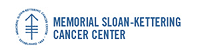Memorial Sloan-Kettering Cancer Center, Dept. of Epidemiology & Biostatistics Working Paper Series

Abstract

Motivation: Array CGH technologies enable the simultaneous measurement of DNA copy number for thousands of sites on a genome. We developed the circular binary segmentation (CBS) algorithm to divide the genome into regions of equal copy number (Olshen {\it et~al}, 2004). The algorithm tests for change-points using a maximal $t$-statistic with a permutation reference distribution to obtain the corresponding $p$-value. The number of computations required for the maximal test statistic is $O(N^2),$ where $N$ is the number of markers. This makes the full permutation approach computationally prohibitive for the newer arrays that contain tens of thousands markers and highlights the need for a faster. algorithm.

Results: We present a hybrid approach to obtain the $p$-value of the test statistic in linear time. We also introduce a rule for stopping early when there is strong evidence for the presence of a change. We show through simulations that the hybrid approach provides a substantial gain in speed with only a negligible loss in accuracy and that the stopping rule further increases speed. We also present the analysis of array CGH data from a breast cancer cell line to show the impact of the new approaches on the analysis of real data.

Availability: An R (R Development Core Team, 2006) version of the CBS algorithm has been implemented in the DNAcopy'' package of the Bioconductor project (Gentleman {\it et~al}, 2004). The proposed hybrid method for the $p$-value is available in version 1.2.1 or higher and the stopping rule for declaring a change early is available in version 1.5.1 or higher.

Genetics

COinS